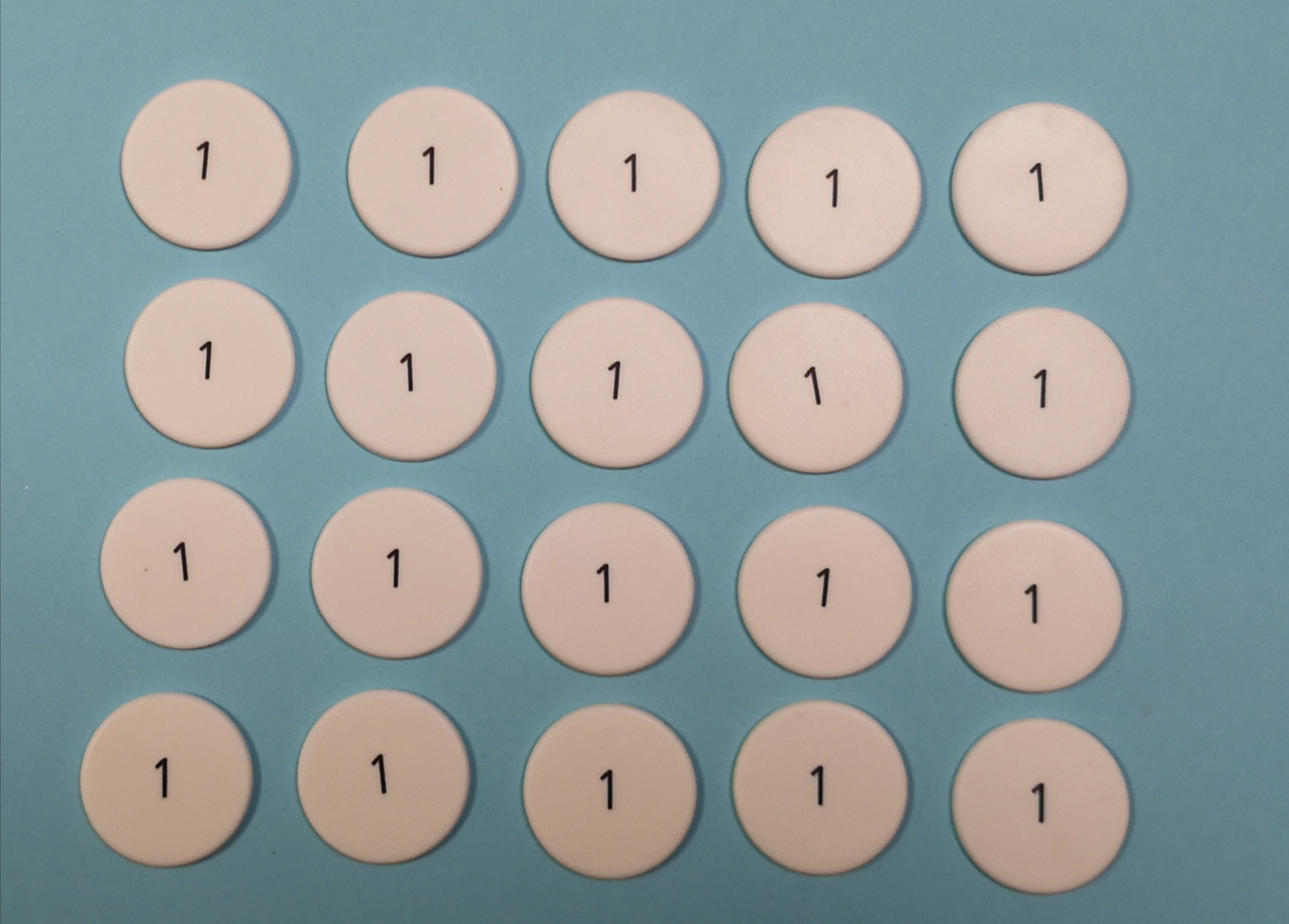SPEND & SAVE! Save up to £50 on over 1000 Early Years resources with code EYSAVING22Blog home
The Blog

# Conceptual vs fluency- learning for multiplication

White Rose Maths explain how to use place value counters to develop conceptual understanding alongside fluency within times tables and multiplication.

# multiplication

Posted on Thursday 31st October 2019

With the introduction of the times table check for Year 4 in June 2020, there has been a huge push in schools towards fluency in multiplication. There are many approaches with a focus on daily practice to develop rapid recall of times table facts.

However, it is also important that children develop a conceptual understanding of multiplication in order to be able to apply their understanding of multiplication to other areas of the curriculum e.g. division, area etc. The use of manipulatives is vital in this understanding so that children can see how multiplication works rather than just being able to recall facts.

### This blog will examine how place value counters can be used to develop conceptual understanding alongside fluency within times tables and then progressing on to more formal methods of multiplication.

Children are introduced to the multiplication sign within Year 2. Over the next 3 years, they are expected to learn all their times tables up to 12 x 12. It is therefore vital that they understand commutativity (numbers can be multiplied in any order and the product will remain the same) and use this to help see links between the times tables.

One way of highlighting commutativity is by using arrays.

Here, we can see an array showing 4 × 5 or 5 × 4. It is important that children can see the array as both 4 groups of 5 as well as 5 groups of 4.This can help them to use the array to predict other multiplications.

Consider how you would adapt the array to show the following:

5×5                  6×4                       4×4                     2×5

Arrays highlight the structure of multiplication as repeated addition and enable children to see how they can use known facts and add or subtract multiples to calculate other multiplications.

e.g. Calculating 10 × 8 – 8 to calculate 9 × 8.

Arrays can also be adapted to consider the link between multiplying ones with multiplying tens and hundreds. Consider replacing the ones in the array shown with tens. What multiplication would be represented? What if the counters were hundreds? Consider showing the multiplication in words alongside manipulatives and calculations to highlight the link even more, e.g. 4 lots of 5 ones =  20 ones , 4 lots of 5 tens = 20 tens, 4 lots of 5 hundreds = 20 hundreds. Children can then regroup the ones, tens and hundreds to see that they are equal to 2 tens, 2 hundreds and 2 thousands.

When children move onto more formal methods of multiplication, encourage them to use place value counters alongside written methods so they understand how and why the method works. In the diagram, we can see how this can be done.

1. Make 4 groups of 123 on the place value grid.

2. Starting with the smallest place value column, calculate how many counters there are in each column. If there are 10, make an exchange.

3. An exchange is shown on the written method by writing a 1 underneath the next column.

As can be seen in the diagrams, using the place value counters highlights what happens when there are 10 in a place value column. Children exchange the counters and then show this in the written method. Using the counters also helps children make connections to exchange in formal addition.

When using counters to understand multiplication, it is vital that teachers choose the numbers carefully. If children end up having to do more than 2 exchanges in one column, this can become unmanageable and accidental mistakes are more likely to be made. The counters are important to support the children in their conceptual understanding of multiplication. Once children understand the method, they should move away from the counters and use the written method supported by their knowledge of times tables. Children can sometimes overly rely on the counters to multiply larger numbers. If a child can use the method effectively to calculate 36 × 5 without counters but struggles with 36 × 7, it is more likely that the child lacks times table knowledge rather than an understanding of the method.

Due to this, it is vital that there is still a focus on developing fluency of times tables. Once children develop the conceptual understanding behind multiplication, they should practice on a daily basis to develop speed and accuracy in their recall. Joining this recall with the use of counters to understand what is happening leads to a deeper understanding of the structures behind formal multiplication.

With thanks to White Rose Maths for writing this blog.